# Unmake the World, Restore Its True Form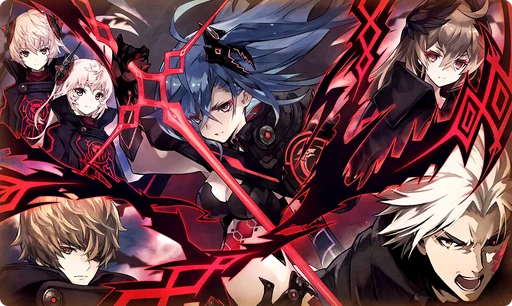How long will we continue to fight? When will we be rewarded? After countless battles, we shine as dully as these rings. "Come. Together we will go...beyond the Void." Do these words form a wish? Are they a plea for atonement? Who could say? They are but words from the lips of those corpses we have trodden to reach this point. Heroes may be called such, but their hearts are no different to that of any other. (Murmurs of the Phantoms Who Have Fallen to the Darkness)

## Stats

#### Simple stats

TypeLvl 1Lvl 30Lvl 40
HP+20+49+60

LevelHP
1+20
2+21
3+22
4+23
5+24
6+25
7+26
8+27
9+28
10+29
LevelHP
11+30
12+31
13+32
14+33
15+34
16+35
17+36
18+37
19+38
20+39
LevelHP
21+40
22+41
23+42
24+43
25+44
26+45
27+46
28+47
29+48
30+49
LevelHP
31+50
32+51
33+52
34+53
35+54
36+55
37+56
38+57
39+58
40+60

## Group skills

### Resentment of the Dark Phantoms

#### Simple stats

TypeLvl 1Lvl 30Lvl 40
HP+100+248+300
PATK+15+33+40
MATK+15+33+40
PDEF+10+24+30
MDEF+10+24+30

#### Detailed stats

LevelHPPATKMATKPDEFMDEF
1+100+15+15+10+10
2+105+15+15+10+10
3+110+16+16+11+11
4+115+16+16+11+11
5+120+17+17+12+12
6+125+18+18+12+12
7+130+18+18+13+13
8+135+19+19+13+13
9+141+20+20+14+14
10+146+20+20+14+14
LevelHPPATKMATKPDEFMDEF
11+151+21+21+15+15
12+156+22+22+15+15
13+161+22+22+16+16
14+166+23+23+16+16
15+171+23+23+17+17
16+176+24+24+17+17
17+182+25+25+18+18
18+187+25+25+18+18
19+192+26+26+19+19
20+197+27+27+19+19
LevelHPPATKMATKPDEFMDEF
21+202+27+27+20+20
22+207+28+28+20+20
23+212+29+29+21+21
24+217+29+29+21+21
25+223+30+30+22+22
26+228+31+31+22+22
27+233+31+31+23+23
28+238+32+32+23+23
29+243+32+32+24+24
30+248+33+33+24+24
LevelHPPATKMATKPDEFMDEF
31+253+34+34+25+25
32+258+34+34+25+25
33+264+35+35+26+26
34+269+36+36+26+26
35+274+36+36+27+27
36+279+37+37+27+27
37+284+38+38+28+28
38+289+38+38+28+28
39+294+39+39+29+29
40+300+40+40+30+30

#### Limit Break

TypeLB1LB2LB3LB4LB5
HP+36+72+108+144+180
PATK+5+9+14+19+24
MATK+5+9+14+19+24
PDEF+4+7+11+14+18
MDEF+4+7+11+14+18

TypeLB5
AGI+8

## Vision abilities

### Zero Mission

#### Ability base

• Slot: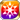Action
• DMG Type: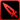Physical
• Element: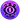Dark

Dark non-type ATK on units within area that increases according to own PDEF (Strong vs Light) [Range: 4, Area: Square (3), Height Range: 3]

Skill Effect
TypeMinMax
Attack80%120%
• Charges: 1
• Jewel Cost: 55
• Height: 3
• Select Range: Diamond
• Range: 0-4
• Select Scope: Square
• Scope: 1

#### Ability max

• Slot:Action
• DMG Type:Physical
• Element:Dark

Dark non-type attack on units within area that increases according to own PDEF (Strong vs Light) [Range: 4, Area: Square (3), Height Range: 3]

Skill Effect
TypeMinMax
Attack168%168%
• Charges: 1
• Jewel Cost: 55
• Height: 3
• Select Range: Diamond
• Range: 0-4
• Select Scope: Square
• Scope: 1

### Zero Mission

#### Ability base

• Slot:Action
• DMG Type: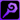Magical
• Element:Dark

Dark non-type ATK on units within area (Strong vs Light) [Range: 4, Area: Square (3), Height Range: 3]

Skill Effect
TypeMinMax
Attack80%120%
• Charges: 1
• Jewel Cost: 55
• Height: 3
• Select Range: Diamond
• Range: 0-4
• Select Scope: Square
• Scope: 1

#### Ability max

• Slot:Action
• DMG Type:Magical
• Element:Dark

Dark non-type ATK on units within area (Strong vs Light) [Range: 4, Area: Square (3), Height Range: 3]

Skill Effect
TypeMinMax
Attack168%168%
• Charges: 1
• Jewel Cost: 55
• Height: 3
• Select Range: Diamond
• Range: 0-4
• Select Scope: Square
• Scope: 1

### Zero Mission

#### Ability base

• Slot:Action
• DMG Type:Magical
• Element:Dark

Dark non-type ATK on units within area (Strong vs Light) [Range: 4, Area: Square (3), Height Range: 3]

Skill Effect
TypeMinMax
Attack80%120%
• Charges: 1
• Jewel Cost: 55
• Height: 3
• Select Range: Diamond
• Range: 0-4
• Select Scope: Square
• Scope: 1

#### Ability max

• Slot:Action
• DMG Type:Magical
• Element:Dark

Dark non-type ATK on units within area (Strong vs Light) [Range: 4, Area: Square (3), Height Range: 3]

Skill Effect
TypeMinMax
Attack168%168%
• Charges: 1
• Jewel Cost: 55
• Height: 3
• Select Range: Diamond
• Range: 0-4
• Select Scope: Square
• Scope: 1

### Zero Mission

#### Ability base

• Slot:Action
• DMG Type:Magical
• Element:Dark

Dark non-type ATK on units within area (Strong vs Light) [Range: 4, Area: Square (3), Height Range: 3]

Skill Effect
TypeMinMax
Attack80%120%
• Charges: 1
• Jewel Cost: 55
• Height: 3
• Select Range: Diamond
• Range: 0-4
• Select Scope: Square
• Scope: 1

#### Ability max

• Slot:Action
• DMG Type:Magical
• Element:Dark

Dark non-type ATK on units within area (Strong vs Light) [Range: 4, Area: Square (3), Height Range: 3]

Skill Effect
TypeMinMax
Attack168%168%
• Charges: 1
• Jewel Cost: 55
• Height: 3
• Select Range: Diamond
• Range: 0-4
• Select Scope: Square
• Scope: 1

### Zero Mission

#### Ability base

• Slot:Action
• DMG Type:Physical
• Element:Dark

Dark non-type ATK on units within area (Strong vs Light) [Range: 4, Area: Square (3), Height Range: 3]

Skill Effect
TypeMinMax
Attack80%120%
• Charges: 1
• Jewel Cost: 55
• Height: 3
• Select Range: Diamond
• Range: 0-4
• Select Scope: Square
• Scope: 1

#### Ability max

• Slot:Action
• DMG Type:Physical
• Element:Dark

Dark non-type ATK on units within area (Strong vs Light) [Range: 4, Area: Square (3), Height Range: 3]

Skill Effect
TypeMinMax
Attack168%168%
• Charges: 1
• Jewel Cost: 55
• Height: 3
• Select Range: Diamond
• Range: 0-4
• Select Scope: Square
• Scope: 1

### Zero Mission

#### Ability base

• Slot:Action
• DMG Type:Physical
• Element:Dark

Dark non-type ATK on units within area (Strong vs Light) [Range: 4, Area: Square (3), Height Range: 3]

Skill Effect
TypeMinMax
Attack80%120%
• Charges: 1
• Jewel Cost: 55
• Height: 3
• Select Range: Diamond
• Range: 0-4
• Select Scope: Square
• Scope: 1

#### Ability max

• Slot:Action
• DMG Type:Physical
• Element:Dark

Dark non-type ATK on units within area (Strong vs Light) [Range: 4, Area: Square (3), Height Range: 3]

Skill Effect
TypeMinMax
Attack168%168%
• Charges: 1
• Jewel Cost: 55
• Height: 3
• Select Range: Diamond
• Range: 0-4
• Select Scope: Square
• Scope: 1

### Limit Reached Bonus

#### Ability base

• Slot:Support

Revealed after Limit Reached. Raises AGI after appearing on map.

#### Ability max

• Slot:Action

Raises AGI after appearing on map.